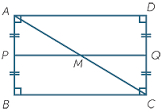# A rectangle 12

A rectangle has a width of 7.2 cm shorter than its length. The perimeter of this rectangle is 22.8 cm. Write the width-to-length ratio of this rectangle in the simplest form and show how you solve the problem.

r =  7:31

### Step-by-step explanation:Did you find an error or inaccuracy? Feel free to write us. Thank you!

Tips for related online calculators
Check out our ratio calculator.
Do you have a linear equation or system of equations and looking for its solution? Or do you have a quadratic equation?
Do you want to convert length units?

#### Grade of the word problem:

We encourage you to watch this tutorial video on this math problem: